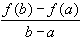#Interactive Real Analysis

Next | Previous | Glossary | Map

## 6.5. Differentiable Functions

### Examples 6.5.10(b):

If f is differentiable on R and | f'(x) |M for all x, then | f(x) - f(y) |M | x - y | for all numbers x, y.
Functions that satisfy the inequality
| f(x) - f(y) |M | x - y |
for some constant M are called Lipschitz functions. Using those terms, we have to prove that if f is differentiable and uniformly bounded then it is a Lipschitz function.

Take any two number a, b. By the mean value theorem we know that there exists an x in (a, b) such that:

f'(x) =Taking absolute values on both sides and moving the denominator to the other side we have

| f(b) - f(a) | = | f'(x) | | b - a |
Since f'(x) is uniformly bounded by M, we therefore have
| f(b) - f(a) |M | b - a |
But that is exactly what we wanted to prove.
Next | Previous | Glossary | Map